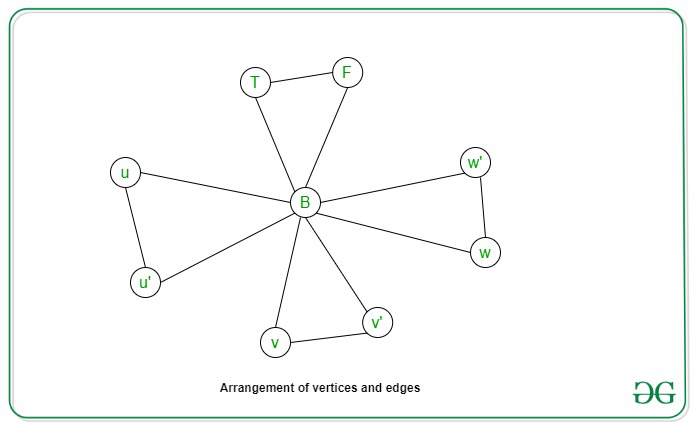# 3-coloring is NP Complete

• Difficulty Level : Hard
• Last Updated : 01 Oct, 2020

Prerequisite: NP-Completeness, Graph Coloring

Graph K-coloring Problem: A K-coloring problem for undirected graphs is an assignment of colors to the nodes of the graph such that no two adjacent vertices have the same color, and at most K colors are used to complete color the graph.

Problem Statement: Given a graph G(V, E) and an integer K = 3, the task is to determine if the graph can be colored using at most 3 colors such that no two adjacent vertices are given the same color.

Explanation:
An instance of the problem is an input specified to the problem. An instance of the 3-coloring problem is an undirected graph G (V, E), and the task is to check whether there is a possible assignment of colors for each of the vertices V using only 3 different colors with each neighbor colored differently. Since an NP-Complete problem is a problem which is both in NP and NP-hard, the proof for the statement that a problem is NP-Complete consists of two parts:

1. The problem itself is in NP class.
2. All other problems in NP class can be polynomial-time reducible to that.(B is polynomial-time reducible to C is denoted as B ≤ PC)

If the 2nd condition is only satisfied then the problem is called NP-Hard.

But it is not possible to reduce every NP problem into another NP problem to show its NP-Completeness all the time. Therefore, to show a problem is NP-Complete, then proof that the problem is in NP and any NP-Complete problem is reducible to that i.e., if B is NP-Complete and B≤PC then for C in NP, then C is NP-Complete. Thus, it can be concluded that the Graph K-coloring Problem is NP-Complete using the following two propositions:

3-coloring problem is in NP:
If any problem is in NP, then, given a certificate, which is a solution to the problem and an instance of the problem (A graph G(V, E) and an assignment of the colors {c1, c2, c3} where each vertex is assigned a color from this three colors {c1, c2, c3}), then it can be verified (Check whether the solution given is correct or not) that the certificate in polynomial time. This can be done in the following way:

For each edge {u, v} in graph G verify that the color c(u) != c(v)

Hence, the assignment can be checked for correctness in the polynomial-time of the graph with respect to its edges O(V+E).

3-coloring problem is NP-Hard:
In order to prove that the 3-coloring problem is NP-Hard, perform a reduction from a known NP-Hard problem to this problem. Carry out a reduction from which the 3-SAT problem can be reduced to the 3-coloring problem. Let us assume that the 3-SAT problem has a 3-SAT formula of m clauses on n variables denoted by x1, x2, …, xn. The graph can then be constructed from the formula in the following way:

1. For every variable xi Construct a vertex vi In the graph and a vertex vi’ denoting the negation of the variable xi.
2. For each clause c in m, add 5 vertices corresponding to values c1, c1, …, c5.
3. Three vertices of different colors are additionally added to denote the values True, False, and Base (T, F, B) respectively.
4. Edges are added among these three additional vertices T, F, B to form a triangle.
5. Edges are added among the vertices vi and vi’ and Base (B) to form a triangle.

The following constraints are true for graph G:

1. For each of the pairs of vertices vi and vi’, either one is assigned a TRUE value and the other, FALSE.
2. For each clause c in m clauses, at least one of the literal has to hold TRUE value for the value to be true.

A small OR- gadget graph therefore can be constructed for each of the clause c = (u V v V w) in the formula by input nodes u, v, w, and connect output node of gadget to both False and Base special nodes.

Let us consider the formula f = (u’ V v V w’) AND (u V v V w’)Now the reduction can be proved by the following two propositions:
Let us assume that the 3-SAT formula has a satisfying assignment, then in every clause, at least one of the literals xi has to be true, therefore, the corresponding vi can be assigned to a TRUE color and vi’ to FALSE. Now, extending this, for each clause the corresponding OR-gadget graph can be 3-colored. Hence, the graph can be 3-colored.

Let us consider that the graph G is 3-colorable, so if the vertex vi is assigned to the true color, correspondingly the variable xi is assigned to true. This will form a legal truth assignment. Also, for any clause Cj = (x V y V z), it cannot be that all the three literals x, y, z are False. Because in this case, the output of the OR-gadget graph for Cj has to be colored False. This is a contradiction because the output is connected to Base and False. Hence, there exists a satisfying assignment to the 3-SAT clause.

Conclusion: Therefore, 3-coloring is an NP-Complete problem.

My Personal Notes arrow_drop_up
Recommended Articles
Page :